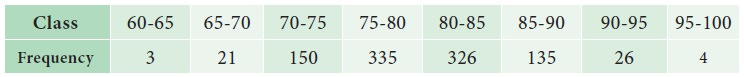Home | | Statistics 11th std | Fitting of Binomial, Poisson and Normal distributions

# Fitting of Binomial, Poisson and Normal distributions

There are many probability distributions of which some can be fitted more closely to the observed frequency of the data than others, depending on the characteristics of the variables.

Fitting of Binomial, Poisson and Normal distributions

## Introduction

Fitting of probability distribution to a series of observed data helps to predict the probability or to forecast the frequency of occurrence of the required variable in a certain desired interval.

There are many probability distributions of which some can be fitted more closely to the observed frequency of the data than others, depending on the characteristics of the variables. Therefore one needs to select a distribution that suits the data well.

## Fitting of Binomial Distribution

When a Binomial distribution is to be fitted to an observed data the following procedure is adopted:-### Example 10.34

A set of three similar coins are tossed 100 times with the following resultsFit a binomial distribution and estimate the expected frequencies.

### Solution :### Solution :## Fitting of Poisson Distribution

When a Poisson distribution is to be fitted to an observed data the following procedure is adopted:### Example 10.35

The following mistakes per page were observed in a bookFit a Poisson distribution and estimate the expected frequencies.

### Solution:### Result:## Fitting of Normal Distribution

In fitting a Normal distribution to the observed data, given in class intervals, we follow the following procedure:-### Example 10.36

Find expected frequencies for the following data, if its calculated mean and standard deviation are 79.945 and 5.545.### Solution:

Given ╬╝= 79.945, Žā = 5.545, and N = 1000

Hence the equation of Normal curve fitted to the data isTheoretical Normal frequencies can be obtained as follows:Study Material, Lecturing Notes, Assignment, Reference, Wiki description explanation, brief detail
11th Statistics : Chapter 10 : Probability Distributions : Fitting of Binomial, Poisson and Normal distributions |